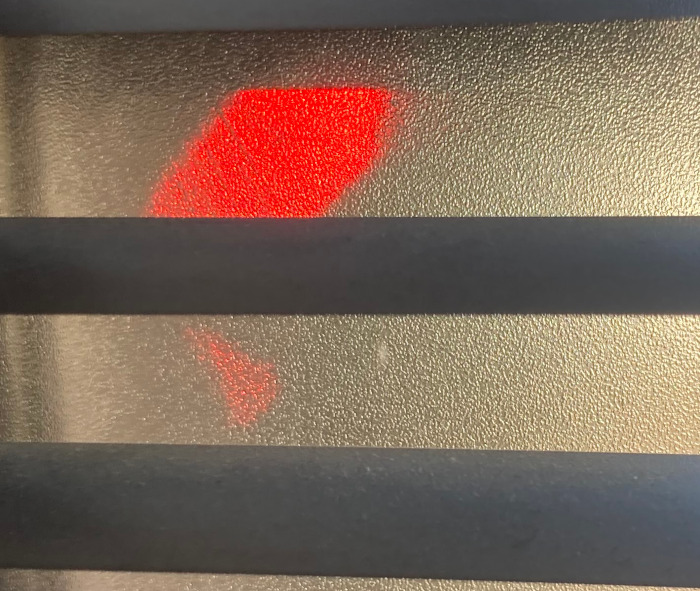# Trying to explain light transmission through a privacy film

25 Apr 2021

A few days ago I was looking at the glass door of the house and there was a red shine.After some investigation, I figured out it was not a laser microphone, but just a standing car. The sun hit the plastic of its light perfectly to focus it on the door. After looking a little more at it, I found it was neither diffuse nor just clear transmission. The light was only visible at a small angle of approximately 20 degrees around the direct (and occluded) line of sight to the light source.

I didn’t understand what was happening so started a little project to see what I can figure out. The door has a grainy privacy film applied. The assumption is, that the grains are the suspect for the scattering.

## A model

The obvious approach to this is to look at some ideas of computer-graphics. Microfacet models are used there to describe the reflection when light hits a surface. In ignorance of the involved scales, we will try to do something similar, but we don’t look at microfacets. We look at micro grains.

A grainy film is a kind of complicated thing so let’s assume there is just a layer of single grains between air. So we just look at a single one. Even this single grain is not that simple, so lets assume it is simple and a uniform perfect sphere. Like everything transparent it has a reflective index $n > 1$. Lets look at how some light rays interact with such a sphere:We see that central rays go through the sphere without any effect and all other rays are scattered to some degree.

## Simulating it

I tried to calculate this by hand and it turns out the math is rather complicated so I turned to numerical simulation!

Simulation is rather easy. We just do what happens in the image above.

• We generate some rays and look where they enter the grain
• Calculate the refracted light direction inside the grain
• Trace the ray in this direction till it leaves the grain
• Look at the scattered direction

Due to the symmetry of a sphere we only need to look at a single line of incoming rays and many calculations are less complicated. After some more thinking we can figure out, that the radius of the sphere does not matter at all and we set it to $1$.

To do all this I wrote the following C code:

for(int i = 0; i != NUM_SAMPLES; i++){
/* Don't take samples at x = 1. This would need more careful handling of edgecases */
float x                = 1.0f*i/NUM_SAMPLES;
vec3  ray_incoming     = V3(0, 0, 1);
vec3  ray_incoming_hit = V3(x, 0, -sqrtf(1-sq(x)));
vec3  normal_incoming  = normalize(ray_incoming_hit);
vec3  ray_internal     = refract(ray_incoming, normal_incoming, 1.0f, n);
vec3  ray_outgoing_hit = ray_hit(ray_incoming_hit, ray_internal);
vec3  normal_outgoing  = normalize(ray_outgoing_hit);
vec3  inormal_outgoing = V3(-normal_outgoing.x, -normal_outgoing.y, -normal_outgoing.z);
vec3  ray_outgoing     = refract(ray_internal, inormal_outgoing, n, 1.0f);
float angle_outgoing   = 3.141592-acos(-ray_outgoing.z);

fprintf(outfile, "%f\n", angle_outgoing);
}


To calculate the refraction direction we have to use Snell’s-law. There is a rather convenient form of it derived in Wikipedia:

vec3 refract(vec3 dir, vec3 normal, float n_in, float n_out){
float r = n_in/n_out;
float c = -dot(normal, dir);
float nfac = r*c-sqrt(1-sq(r)*(1-sq(c)));
return normalize(V3(r*dir.x+nfac*normal.x,
r*dir.y+nfac*normal.y,
r*dir.z+nfac*normal.z));
}


To get the point where the ray leaves the grain again we simply solve $(\vec o+t\vec d)^2 = 1^2$, where $\vec o$ is the origin and $\vec d$ the direction of the ray.

You can see the complete code on GitHub.

## ResultsIn the image above I calculated the distribution of scattered light directions for some different values of the refractive index. For $n$ near $1$ we get a sharp distribution, but for $n=1.5$ the distribution become rather wide.

Looking at many simulations by hand is rather tedious so we need to quantify the result of such a distribution. Similar to physics we will look at the angle where the distribution falls of to half of its maximum value. This is easy to calculate and we get:## Problem solved?

So looking at the graph and assuming the 20-degree angle from above, there is a solution. The privacy film consists of grains with a refractive index of $n\approx 1.25$ and we are done!

Sadly if we look at this result a little more, this is all rather unlikely to be correct. Optical glass has a refractive index $n\approx 1.52$. I don’t think this 20-year-old privacy film (most likely from IKEA) has a lower refractive index than that.

Most likely we need to examine the parts between the grains and even the microsurface of the grains themself. Maybe we should look at the surface between the glass and the grains some more. We assumed the door is just air for now. This would most probably need usage of advanced measurement tools and removing some of the film from the door and I will do neither of these things at the moment!

We only learned of a simple model for light transmission through a layer of grains and how to calculate that. This has to be enough for now.